# GSEB Solutions Class 10 Maths Chapter 7 Coordinate Geometry Ex 7.4

Gujarat Board GSEB Solutions Class 10 Maths Chapter 7 Coordinate Geometry Ex 7.4 Textbook Questions and Answers.

## Gujarat Board Textbook Solutions Class 10 Maths Chapter 7 Coordinate Geometry Ex 7.4

Question 1.
Determine the ratio in which the line 2x + y – 4 = 0 divides the line segment joining the points A(2, -2) and B(3, 7).
Solution:
Let the line 2x + y – 4 = 0 divide the line segment joining the points A(2, -2) and B(3, 7) in the ratio λ : 1. Let the point of intersection be P. Then,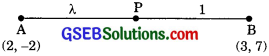Coordinates of P are given by
P$$\left[\frac{(\lambda)(3)+(1)(2)}{\lambda+1}, \frac{(\lambda)(7)+(1)(-2)}{\lambda+1}\right]$$
⇒ $$\left(\frac{3 \lambda+2}{\lambda+1}, \frac{7 \lambda-2}{\lambda+1}\right)$$
∴ P lies on the line 2x + y – 4 = 0
∴ 2 $$\left(\frac{3 \lambda+2}{\lambda+1}\right)+\left(\frac{7 \lambda-2}{\lambda+1}\right)$$ – 4 = 0
⇒ 2(3λ + 2) + (7λ – 2) – 4(λ + 1) = 0
6λ + 4 + 7λ – 2 – 4λ – 4 = 0
9λ – 2 = 0
Hence, the required ratio is 2 : 9.Question 2.
Find a relation between x and y if the points (x, y), (1, 2) and (7, 0) are collinear.
Solution:
If the given points are collinear, then the area of the triangle with these points as vertices will be zero.
$$\frac {1}{2}$$[x(2 – 0) + 1(0 – y) + 7(y – 2)]= 0
= $$\frac {1}{2}$$ [2x – y + 7y – 14] = 0
= [2x + 6y – 14] = 0
2x + 6y – 14 = 0
x + 3y – 7 = 0

Question 3.
Find the centre of a circle passing through the points (6, -6), (3, -7) and (3, 3).
Solution:
Let the given points be A(6, -6), B(3, -7) and C(3, 3).
Let the centre of the circle be O(x, y).
Then, OA = OB = OC [radii of circle]
(OA)2 = (OB)2 = (OC)2 ……….(1)
From (1), we have .
OA2 = OB2
⇒ (x-6)2 +(y+6)2 = (x-3)2 + (y+ 7)2
⇒ x2 – 12x + 36 + y2 + 12y + 36
⇒ x2 – Gx + 9 + y2 + 14y + 49
⇒ 6x + 2y = 14
⇒ 3x + y = 7
Again, we have
(OB)2 = (OC)2
(y + 7)2 = (y – 3)2
y2 + 49 + 14y = y2 + 9 – 6y
20 y = 9 – 49
20 y = -40
y = -2
Putting the value ofy in (2), we get x = 3.
Hence, centre of a circle = 0(3, -2).Question 4.
The two opposite vertices of a square are (-1, 2) and (3, 2). Find the coordinates of the other two vertices.
Solution:
Let A(-1, 2) and C(3, 2) be the two opposite vertices of a square ABCD. Let B(x, y) be the unknown vertex.
Then, AB = BC
AB2 = BC2
⇒ (x + 1)2 + (y – 2)2 = (x – 3)2 + (y – 2)2
⇒ x2 + 2x + 1 + y2 – 4y + 4
⇒ x2 – 6x + 9 + y2 – 4y + 4
⇒ 8x = 8
x = 1
Also, AB2 + BC2 = AC2
(∴ ∠B = 90º and therefore using Pythagoras Theorem]
(x + 1)2 + (y – 2)2 + (x – 3)2 + (y – 2)2
= (3 + 1)2 + (2 – 2)2
=x2 + 2x + 1 + y2 – 4y + 4 + x2 – 6x + 9 + y2 – 4y + 4 = 16
= 2x2 + 2y2 – 4x – 8y + 2 = 0
= x2 + y2 – 2x – 4y + 1 = 0
[Dividing throughout by 2]
Putting x = 1, we get
1 + y2 – 2 – 4y + 1 = 0
y(y – 4) = 0
y = 0, 4
Hence, the other vertices are (1, 0) and (1, 4).Question 5.
The Class X students of a secondary school in Krishna Nagar have been allotted a rectangular plot of a land for their gardening
activity. Saplings of Gulmohar are planted on the boundary at a distance of 1 metre from each other. There is triangular grassy lawn in the plot as shown in the figure. The students are to sow seeds of the flowering plants on the remaining area of the plot.
(i) Taking A as origin, find the coordinates of the vertices of the triangle.
(ii) What will be the coordinates of the vertices of ∠PQR if C is the origin?
Also, calculate the area of the triangle in these cases. What do you observe?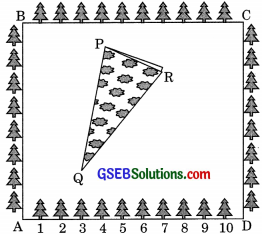Solution:
(i) Taking A as origin, AD and AB as coordinate axes.
The coordinates of P are (4, 6).
The coordinates of Q are (3, 2).
The coordinates of R are (6, 5).
Area of ΔPQR
= $$\frac {1}{2}$$ [x1(y2 – y3) + x2(y3 – y1) + x3(y1 – y2)]
= $$\frac {1}{2}$$ [4(2 – 5) + 3(5 – 6) + 6(6 – 2)]
= $$\frac {1}{2}$$ [4(-3) + 3(-1) + 6(4)]
= $$\frac {1}{2}$$ [-12 – 3 + 24]
= $$\frac {9}{4}$$ square units.

(ii) Taking C a origin, CB and CD as coordinate axes.
The coordinates of P are (12, 2)
The coordinates of Q are (13, 6)
The coordinates of R are (10, 3)
Area of ΔPQR
= $$\frac {1}{2}$$[(y2 – y3) + x2(y3 – y1) + x3(y1 – y1)]
= $$\frac {1}{2}$$[12(6 – 3) + 13(3 – 2) + 10(2 – 6)]
= $$\frac {1}{2}$$[12 x 3 x + 13 + 1 + 10(-4)]
= $$\frac {1}{2}$$[36 + 13 – 40]
= $$\frac {1}{2}$$(9) = $$\frac {9}{4}$$ square units.
Thus we observe: Areas are the same in both the cases.Question 6.
The vertices of a tABC are A(4, 6), B(1, 5) and C(7, 2). A line is drawn to intersect sides AB and AC at D and E respectively, such that $$\frac {AD}{AB}$$ = $$\frac {AE}{AC}$$ = $$\frac {1}{4}$$ calculate the area of the
ΔADE and compare it with the area of ΔABC.
Solution:
We have,
$$\frac {AD}{AB}$$ = $$\frac {AE}{AC}$$ = $$\frac {1}{4}$$
⇒ $$\frac {AD}{AB}$$ = $$\frac {AE}{AC}$$ = 4
⇒ $$\frac{\mathrm{AD}+\mathrm{DB}}{\mathrm{AD}}$$ = $$\frac{\mathrm{AE}+\mathrm{EC}}{\mathrm{AE}}$$ = 4
[Taking reciprocals of both sides]
⇒ 1 + $$\frac {DB}{AD}$$ = 1 + $$\frac {EC}{AE}$$ = 4
⇒ $$\frac {DB}{AD}$$ = $$\frac {EC}{AE}$$ = 3
⇒ $$\frac {DB}{AD}$$ = $$\frac {AE}{EC}$$ = $$\frac {1}{3}$$
⇒ AD : DB = AE : EC = 1 : 3
D and E divide AB and AC respectively in the ratio 1 : 3.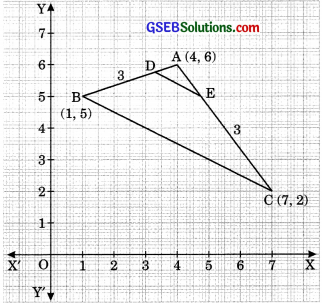So, the coordinates of D and E are
D$$\left(\frac{1+12}{1+3}, \frac{5+18}{1+3}\right)$$ = D$$\left(\frac{13}{4}, \frac{23}{4}\right)$$
and E $$\left(\frac{7+12}{1+3}, \frac{2+18}{1+3}\right)$$ = E$$\left(\frac{19}{4}, 5\right)$$ respectively.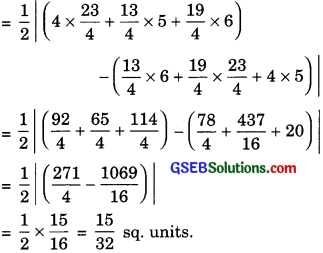Now, Area of ΔABC
= $$\frac {1}{2}$$[(4 x 5 + 1 x 2 + 7 x 6) – (1 x 6 + 7 x 5 + 4 x 2)]
= $$\frac {1}{2}$$[(20 + 2 + 42) – (6 + 35 + 8)]
= $$\frac {1}{2}$$[64 – 49] = $$\frac {15}{2}$$ sq. units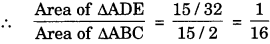Hence, ar(ΔADE) : ar(ΔABC) = 1 : 16.Question 7.
Let A(4, 2), B(6, 5) and C(1, 4) be the vertices of ΔABC.
(i) The median from A meets BC at D. Find the coordinates of the point D.
(ii) Find the coordinates of the point P on AD such that AP: PD = 2: 1.
(iii) Find the coordinates of points Q and R on medians BE and CF respectively such that
BQ : QE = 2 : 1 and CR : RF = 2 : 1.
(iv) What do you observe?
(v) If A(x1, y1), B(x2, y2) and C(x3, y3) are the vertices of ΔABC, find the coordinates of the centroid of the triangle.
Solution:
(i) The median from A meets BC at D.
∴ D is the mid-point of BC. Then coordinates of D are
⇒ D $$\left(\frac{6+1}{2}, \frac{5+4}{2}\right)$$ [Using mid-point formula]
⇒ D $$\left(\frac{7}{2}, \frac{9}{2}\right)$$

(ii) Co-ordinate of P are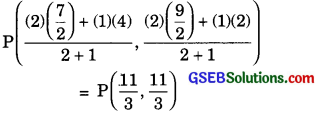(iii) Now coordinates of E are E $$\left(\frac{4+1}{2}, \frac{2+4}{2}\right)$$
⇒ E = $$\left(\frac{5}{2}, 3\right)$$
Coordinates of Q are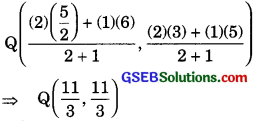And coordinates of F are
F = $$\left(\frac{4+6}{2}, \frac{2+5}{2}\right)$$
F = $$\left(5, \frac{7}{2}\right)$$
Coordinates of R are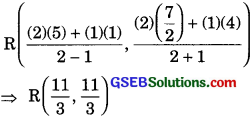(iv) We observe that P, Q, R are the same point.
(v) We know that the centroid of a triangle divides each median of the triangle form the vertex in the ratio 2 : 1. Let D be the mid-point of BC.
Then,
Coordinates of D is
= D$$\left(\frac{x_{2}+x_{3}}{2}, \frac{y_{2}+y_{3}}{2}\right)$$
Let G(x, y) be the centroid of MEC. Then, G will divide AB internally in the ratio 2: 1.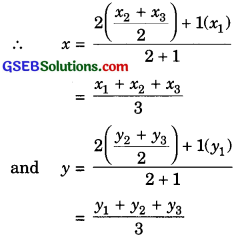Hence, the co-ordinates of the centroid are given by
$$\left(\frac{x_{1}+x_{2}+x_{3}}{3}, \frac{y_{1}+y_{2}+y_{3}}{3}\right)$$Question 8.
ABCD is a rectangle formed by the points A(- 1, -1), B(-i, 4), C(5, 4) and D(5, -1). P, Q, R and S are the mid-points of AB, BC, CD and DA respectively. Is the quadrilateral PQRS a square? a rectangle? or a rhombus? JustifSr your answer.
Solution:
We have, following given points
A(-1, -1), B(-1, 4), C(5, 4) and D(5, -1).
Therefore, coordinates of P are
p$$\left(\frac{-1-1}{2}, \frac{-1+4}{2}\right)$$
$$\left(-1, \frac{3}{2}\right)$$
Coordinates of Q are
Q = $$\left(\frac{-1+5}{2}, \frac{4+4}{2}\right)$$
⇒ Q(2,4)
Coordinates of R are
= R $$\left(\frac{5+5}{2}, \frac{-1+4}{2}\right)$$
⇒ R $$\left(5, \frac{3}{2}\right)$$
And, coordinates of S are
= S$$\left(\frac{-1+5}{2}, \frac{-1-1}{2}\right)$$
S(2,-1)
Now,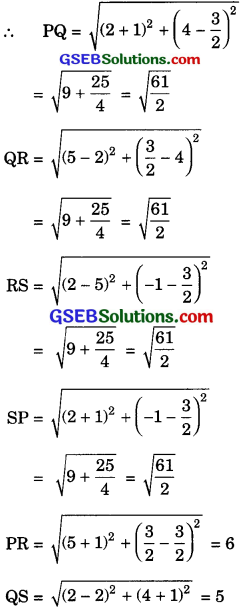QS = $$\sqrt{(2-2)^{2}+(4+1)^{2}}$$ = 5
We see that
PR = QR = RS = SP
Therefore, PQRS is a rhombus.